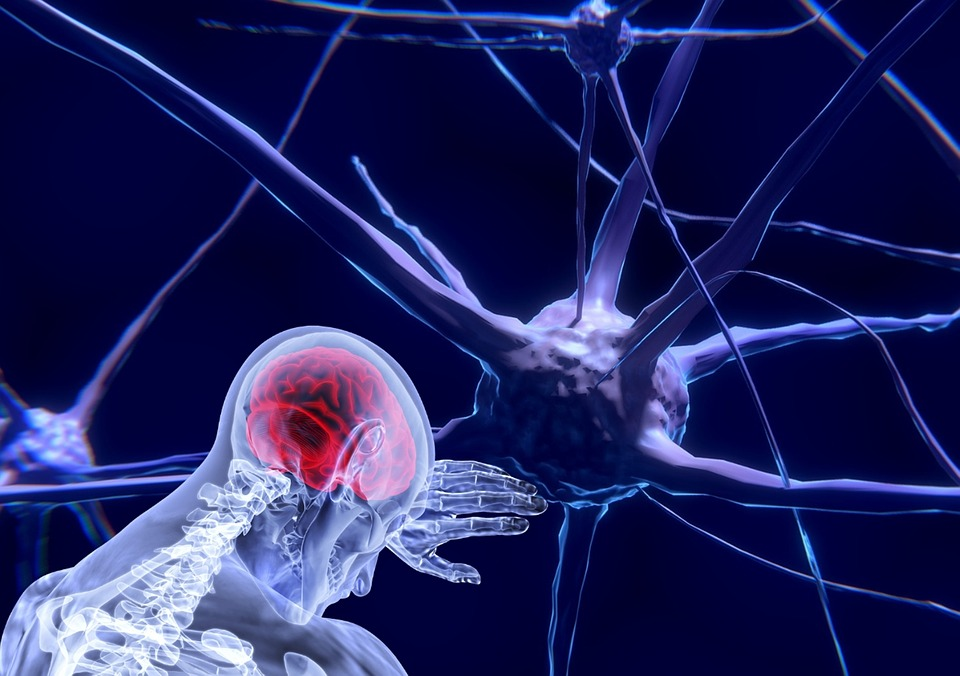## TO THE DEEPEST: Convolutional Neural NetworksConvolutional Neural Networks (CNN), another Deep Learning architecture, is explicitly used to differentiate. They are a derivative of multilayer sensors inspired by biology. Convolutional neural networks consist of many layers that can be trained. Each layer has three layers: the feature pooling layer, the filter bank layer, and the nonlinear layer. There are many kernels for different feature extraction in the filter bank layer. In the pooling layer, each feature map obtained is handled separately.

This algorithm consists of different layers, which capture and classify the features in the visuals with other operations. The visual, which passes through these layers, Convolutional Layer, Pooling, and Fully Connected, is subjected to different processes and reaches the consistency to enter the Deep Learning Model. Creating Convolutional Neural Networks (CNN) Models takes a lot of effort in data preprocessing compared to classical Machine Learning algorithms, as we deal with unstructured (irregular) data.

The Convolutional is the first layer that handles the image in Convolutional Neural Networks (CNN) algorithms. As it is known, images are matrices consisting of pixels with specific values ​​. In the convolution layer, a filter smaller than the original image size hovers over the image and tries to capture particular features from these images.

The parameters learned in Convolutional Neural Networks (CNN) algorithms are the values ​​in these filters. The model constantly updates these values ​​and begins to detect features even better. Also, images can be sharpened or blurred; edge detection can be made with some known filters.

• Stride

The Stride value is a value that can be changed as a parameter in Convolutional Neural Networks (CNN) models. This value determines how many pixels the filter will slide over the main image.

• PoolingLike the Convolutional Layer, the Pooling layer also aims to reduce dimensionality. In this way, both the required processing power is reduced, the unnecessary caught features are ignored, and more essential elements are focused on.

This kernel still hovers over the image in the Pooling layer, which has a grain as in the Convolutional Layer. But instead of a convolutional operation, it applies the determined Pooling technique this time. In other words, if you are using max Pooling, it takes the most significant value in the area covered by the filter, and if you are applying average Pooling, it takes the average of the values ​​in the filter. This reduces the size and retains essential features.

When applying the filter to an image, the output will be smaller than the original image due to dimensions. The method we can use to prevent this is Padding. During the filling process, zeros are added to the image on all four sides as if it were a frame. Depending on the size of the filter, these zero–added layers can be increased.

• ReLU (Rectified Linear Unit)

ReLU (Rectified Linear Unit) is a nonlinear function that operates as f(x) = max(0,x). For example, a ReLU function that takes the value –25 will output 0, and a function that takes the value 25 will output 25. ReLU, whose main purpose is to eliminate negative values, has a critical position in CNNs.

Nonlinear functions such as ReLU and sigmoid are used to prevent our model from learning negative values ​​or not being able to grasp some features due to these negative values.

There are two different pooling techniques generally used in Convolutional Neural Networks (CNN) models. One of them is Max (Maximum), and the other is Average pooling.

Convolutional Neural Networks (CNN) are applicable to Image Processing, Natural Language Processing, and other Cognitive Tasks.

Like other neural networks, a Convolutional Neural Network (CNN) has an input layer, an output layer, and several hidden layers. This simulates some action in the human visual cortex.

Convolutional Neural Networks (CNN) is a prime example of Deep Learning, where a more complex model is pushing the evolution of Artificial Intelligence (AI) by presenting systems that simulate different biological human brain activity.

If we ask what some of the Convolutional Neural Networks (CNN) architectures that are very well known today are, we can list them as follows:

• LeNet
• ResNet
• AlexNet
• VGGNet